Parenting

# How Reasoning is Important in Mental Calculations

3.2k views

 1 Introduction 2 Shakuntala Devi: The Human Computer 3 Importance of Mathematical Reasoning 4 Conclusion 5 Frequently Asked Questions (FAQs) 6 External References

## Introduction

Can you calculate 340 x 32 under a minute?

Many of us tend to think that being great at math is all about being able to calculate fast. Mental calculation is important.

This perception is reinforced through popular media and entertainment channels where individuals who can mentally calculate fast are labeled geniuses.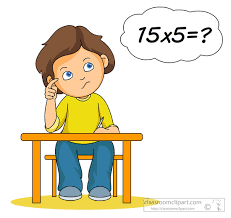Math is much more than calculations. Being able to calculate fast is only a subset of math ability in general.

Mental calculation is used for computing that is based on anyone's understanding and knowledge of mathematical properties and relationships.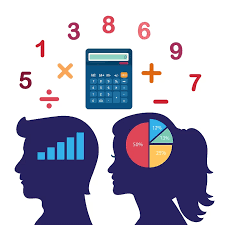Mathematical reasoning is defined as the process of reaching a decision or solution by using critical, creative, and logical thinking.

Reasoning includes understanding the proofs and assessing the arguments. Mental Calculation without mathematical reasoning is irrelevant.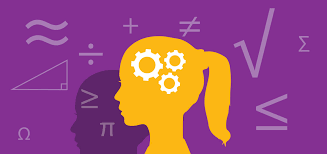Thinking mathematically, exploring, questioning, working systematically, visualizing, explaining, generalizing, justifying, proving, mathematical reasoning are all at the heart of mathematical thinking.

Develop mathematical habits in mind; such as being curious, being collaborative, being resourceful, and resilient.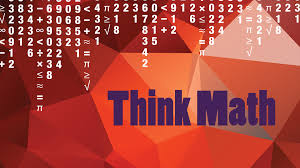### How Reasoning is Important in Mental Calculations-PDF

Cuemath’s learning platform has carefully designed simulations to help the student visualize concepts. This blog will help us understand How Reasoning is Important in Mental Calculations. Here is a downloadable PDF to explore more.

## Shakuntala Devi: The Human Computer

Shakuntala Devi was born on 4th November 1929 in Bangalore, Karnataka. She had unparalleled talent in calculating complex mathematical numbers. She proved her exceptional talent in arithmetic maths through her math shows worldwide at a very tender age.

Her excellent skill has brought her a renowned name as “Human-Computer.” Shakuntala Devi was also an author and showcased her wisdom through books on astrology, puzzles, and mathematics.She made our country proud throughout the world by securing a place in “The Guinness Book of World Records” for her intellectual mathematical talent. A motivational speaker who enlightened the lives of many individuals towards mathematics.

## Importance of Mathematical Reasoning

Students recognize that mathematics makes sense and can be understood with the development of mathematical reasoning.

They learn how to evaluate situations, select problem-solving strategies, draw logical conclusions, develop and describe solutions, and recognize how those solutions can be applied. Mathematical reasoners are able to reflect on solutions to problems and determine whether or not they make sense.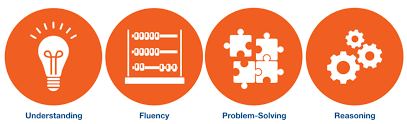Some of the world’s best mathematicians are only average when it comes to mental calculations. Also, the ability to calculate fast is almost never tested on competitive academic or professional exams.

For example, in one of the most competitive exams of the world – the IIT JEE (joint entrance exam) – what is tested is not the ability to calculate fast but the ability to think from first principles.

Given this, the ability to calculate fast is a good-to-have skill, but not a must-have-skill. Children need to be taught something far more fundamental.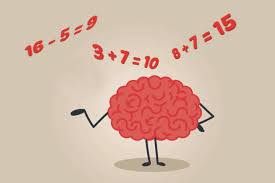Let us illustrate this with an example.

Ritesh* was a Grade-6 child who could calculate exceptionally fast, because he was part of one of these calculation programs where they teach children how to use a mechanical counting device to calculate fast. He could multiply two-digit numbers in his head effortlessly.

During our interaction with him, we posed this question to him: If the price of a book is Rs. 18, what will be the price of twelve such books? In response to this question, he asked us: Should I add these numbers, or should I multiply them?

This is an unfortunate example of what happens when you teach children to calculate mechanically, but not teach them to think or apply.

Ritesh could calculate much faster than an average adult, but he could not tackle even basic applications of those calculation skills.

In such a scenario, one is forced to ask this question. What is the use of teaching the child to calculate using a mechanical device, when there is no thinking involved in that process? How is it any better than using a digital calculator?Here’s another example of the consequence of children being trained to calculate mechanically.

In a Cuemath survey, a group of Grade-6 and Grade-7 children were asked the following question: If all the numbers from 21 to 31 are multiplied, what will be the unit's digit of the product? All these children had been attending various calculation programs for the past many months.

The response of most children to this question was to explicitly carry out the multiplication: 21 x 22 = 462, 462 x 23 = 10626, and so on. However, no student realized that the answer is directly evident: there’s a 30 in the product, so the units digit of the product will be 0.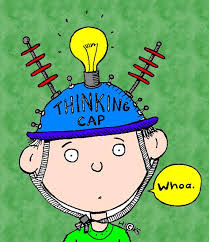Attending these programs makes the children so accustomed to the process of calculation itself that they lose the ability to consider a problem from any other perspective.

## Conclusion

Teach your kid basics and use mental math strategies to improve their skills and to solve complex calculations in head. Studying and practicing math can improve mental calculations.

Through a strong emphasis on fundamentals and exposing children to the logical interconnections between various mathematical ideas, will make children fall in love with math, and hence make them great at thinking and calculating math.

Related Articles
Award-winning math curriculum, FREE for a year